# Multiplication and Division of Algebraic Expressions

Go back to  'Algebra Basics'

## Rules of working with variables

Look at these three examples for a quick recap of the rules of dealing with variables.

\begin{align}3y & = 3 \times y \\ & = y + y + y \\\\ y^3 &= y \times y \times y \\\\ 2y^3 &= 2 \times y \times y \times y \end{align}

## The Rules of Multiplication of Algebraic Terms

### How to Multiply a Monomial by another Monomial?

These examples cover all the rules you’ll need to know for multiplying monomials.

• $$a \times a = a^2$$
• $$a \times b = ab$$
• $$a \times b \times c = abc$$
• $$4a \times c = 4ac$$
• $$ab \times 3{a^2}c = 3{a^3}bc$$
• $$ab \times xy = abxy$$

### How to Multiply a Monomial by a Binomial?

These examples cover all the rules you’ll need to know for multiplying a monomial with a binomial.

• $$y \times (a + b) = ya + yb$$
• $$(a + b) \times c = ac + bc$$
• $$4a \times (3a^2 + b) = 12a^3 + 4ab$$

The monomial term outside the bracket multiplies every term inside the bracket.

### How to Multiply a Binomial by a Binomial?

When multiplying a binomial with another binomial, you will get 4 terms initially, as shown by the arrows in the example below. These terms may simply so the final answer may have fewer terms.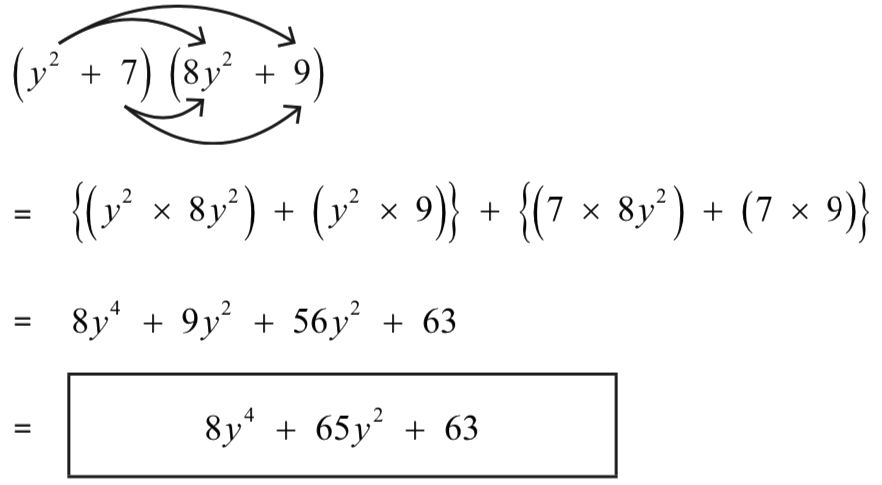## How to Visualise the Multiplication of Algebraic Terms?

Consider the multiplication of a binomial and a monomial:

$a \times (a + b)$

We visualise $$a$$ as the breadth of a rectangle and $$(a + b)$$ as the length. So their product will be the area of this rectangle.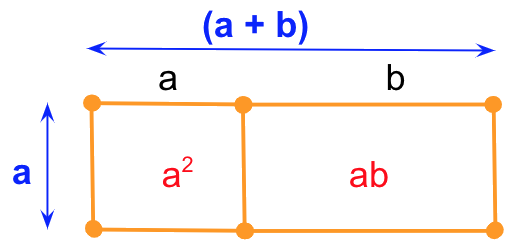So, the area of the rectangle is the sum of all its parts. That is:

$a \times (a + b) = a^2 + ab$

Now let’s visualise the multiplication of a binomial with a binomial:

$(a + c) \times (a + b)$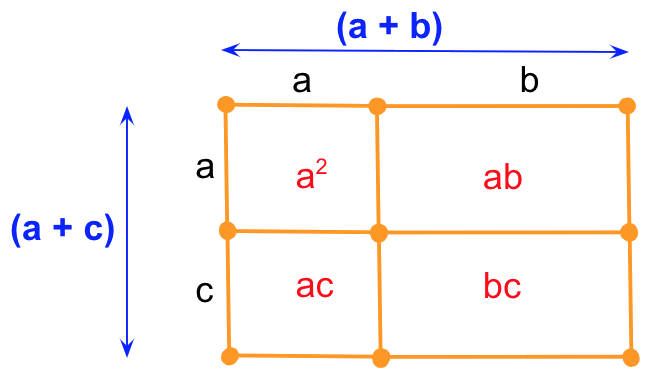So, the area of the rectangle is the sum of all its parts. That is:

$(a + c) \times (a + b) = a^2 + ab + ac + bc$

## The Rules of Division of Algebraic Terms

• $$y \div y = 1$$
• $$5y \div y = 5$$
• \begin{align}4y \div 3y = {4 \over 3} \end{align}
• $$7y^2 \div y = 7y$$ … see a detailed explanation for this rule below.

### How to Divide a Monomial by another Monomial?

Think of division of algebraic terms like you would think of fractions. So, to divide $$9y^5$$ by $$3y^2$$ begin by writing the expression as a fraction: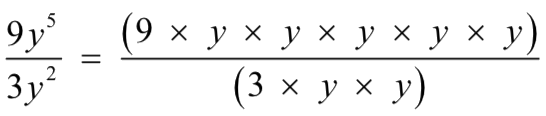Recall the steps to reduce a fraction to its lowest or simplest form. We identify common factors and divide both numerator and denominator by it. We do something similar here.

We see that $$3 \times y \times y$$ is common to both the numerator and denominator.

Cancelling (or technically, dividing both numerator and denominator by) the common term gives us: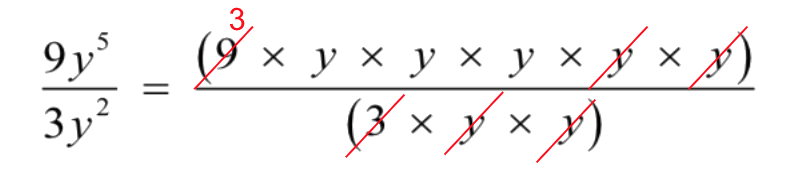So, the answer is $$3y^3$$

### How to Divide a Binomial by a Monomial?

When working with whole numbers, dividing the sum of two numbers by another number is equivalent to dividing each number by the divisor.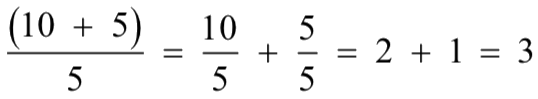Dividing a binomial by a monomial is similar: Let's divide the binomial $$(4y^3 + 8xy)$$ by $$2y.$$

We will divide each term from the binomial by the monomial.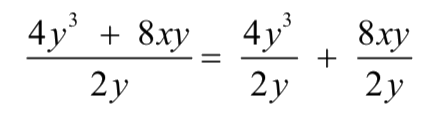This gives us two monomial divisions which we already know how to do. So, the answer is:

$2y^2 + 4x$

## Tips and Tricks to Multiply and Divide Algebraic Expressions

• Watch out for the most common error when multiplying algebraic expressions:
$$2(x + 6)$$ might be wrongly equated to $$2x + 6$$
.

Recall the distributive property of multiplication. All terms inside the bracket need to be multiplied with the multiplier outside the bracket.
So, $$2(x + 6) = 2x + 12$$
A helpful tip is to quickly verify after solving that each term in the bracket has been multiplied correctly.
• Another common error is when terms in the bracket need to be multiplied by a negative term. Remember the negative sign also distributes to all terms in the bracket.
So, $$x - 2(x + 6)$$ might wrongly get written as $$x - 2x + 12$$ instead of $$x - 2x - 12.$$
• When multiplying two polynomials, count the number of terms in each polynomial. Multiply them to know how many terms you should end up with when you multiply those two polynomials.
E.g. When multiplying $$(x + 6)$$ and $$(2y - 3x +1)$$ we have 2 terms in the first polynomial and 3 in the second. So, after multiplying you should get 6 terms. If you have more or less, then check you working.

$$(x + 6)(2y - 3x + 1) = 2xy - 3x^2 + x + 12y - 18x + 6$$